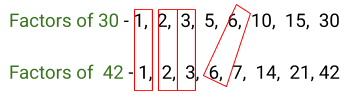In the verge of coronavirus pandemic, we are providing FREE access to our entire Online Curriculum to ensure Learning Doesn't STOP!

# Highest Common Factor

Go back to  'Factors, Multiples, Primes'

## What is the HCF?

HCF stands for Highest Common Factor. Sometimes it is also referred to as the Greatest Common Divisor (GCD).

Factors of a given number are numbers that can perfectly divide that given number

When we consider two numbers, each has its own set of factors. Some of the factors are common. The highest common factor is the common factor and the largest factor of the two numbers.

### Factors of 24 and 48

For example, the factors of 24 and 48 are given below.

24 -  1, 2, 3, 4, 6, 8, 12, 24

48 -  1, 2, 3, 4, 6, 8, 12, 16, 24, 48

The common factors are 1, 2, 3, 6, 8, 12, 24. 24 is the largest of them.

The HCF of 24 and 48 is 24

## How to find the HCF of two or more numbers?

There are 3 methods to calculate the HCF of two numbers,

1. by listing out the factors
2. by prime factorization
3. division method

### 1. By listing out the common multiples

HCF of 30 and 42

Step 1 - List out the factors of each number,

Factors of 30 - 1, 2, 3, 5, 6, 10, 15, 30

Factors of  42 - 1, 2, 3, 6, 7, 14, 21, 42

Step 2 - Circle all the common factorsStep 3 - 6 is the common factor and the greatest one.

Hence the HCF of 30 and 42 is 6.

### 2.  By prime factorization

HCF of 60 and 90

Step 1 - Represent the numbers in the prime factored form

HCF of 60 and 90

Step 1 - Represent the numbers in the prime factored form

60 = 2 x 2 x 3 x 5

90 = 2 x 3 x 3 x 5

Step 2 - HCF is the product of the factors that are common.

HCF of 60 and 90 = 2 x 3 x 5 = 30

### 3. By common division method

HCF of 24 & 60

Step 1 - Find a prime number which is a factor of both numbers. Write this prime number on the left of the two numbers, as shownStep 2 -  Divide both numbers by this prime number, and write the quotients below the numbers.Step 3 - Keep repeating both steps for the quotient obtained.Step 4 - If you’re unable to find a common prime factor, then the HCF will be the product of all the numbers in the left column.

Therefore the HCF of 24 and 60 is 2 x 2 x 3 = 12

Here is how Cuemath students learn to visualise HCF

Experience this in a Cuemath class. Book a Cuemath demo class with a nearby centre

## Tips and tricks to finding HCF

• The listing method works best for the smaller numbers.
For example, listing multiples of 15 & 20 and finding out the least common multiple is easier.
• With larger numbers listing multiples would be a tedious task. It is best to use the prime factorization method.
For example, listing multiples for 150 and 242 would be very tedious. Prime factorization of these numbers would be simpler.
• When you have to find out the LCM or HCF of 3 or more numbers using the common division method would be the best. It gives the flexibility to factorize the numbers simultaneously.

• ## Misconception: Zero is the LCM of any two numbers LCM is the smallest non-zero multiple of two numbers. Non-zero multiples exclude only the number 0 which is technically the very first multiple of any number.

• Misconception: One is the HCF of any two numbers
The highest common factor is the greatest common factor of the two numbers. Well, 1 can be the HCF when two numbers don’t have any common factors other than one. However, when numbers have more than one common factor the greatest number would be the HCF.

## Find HCF and LCM

1. Find LCM of 25 and 63
__________________________

2. Find HCF of 24 and 60
__________________________

3. Find LCM of 99 & 96
__________________________

4. Find HCF and LCM of 44, 64, 96
__________________________

5. Find LCM and HCF of 480 and 720?
__________________________